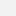# Humboldt-Universität zu Berlin - Statistik

Multivariate Statistical Analysis II (VL)

## Multivariate Statistical Analysis II (VL)Kategorie
Master
Lehrende(r)
Z. Hlavka, J. Mungo, O. Okhrin, J. Hurt

# Course Outline

• Factor analysis is a statistical data reduction technique used to explain variability among observed random variables in terms of fewer unobserved random variables called factors. The observed variables are modeled as linear combinations of the factors, plus "error" terms. The analysis will isolate the underlying factors that explain the data. For factor specification principal component analysis or common factor analysis cab be used which are studied in the MVA 1.
• Canonical correlation analysis tries to establish whether or not there are linear relationships among two sets of variables (covariates and response). It searches vectors a and b such that the random variables a'X and b'Y maximize the correlation• A significant part of the course is devoted to data mining techniques. Classification and Regression Trees (CART) classifies the data to predefined classes using so-called decision trees. By asking only yes/no question dataset is split always into two subgroups. The process is than repeated for each of the resulting subsets until a desired size of the tree is reached. Support Vector Machines (SVM) goes further than CART and splits the data with non-linear decision rule. SVM has showed itself as an efficient tool for credit scoring and insolvency analysis.• An artificial neural network (ANN) is a non-linear statistical data modeling tool which can be used to model complex relationships between inputs and outputs or to find patterns in data. The function f(x) is defined as a composition of other functions g(x), which can further be defined as a composition of other functions. A widely used type of composition is the nonlinear weighted sumThis can be conveniently represented as a network structure, with arrows depicting the dependencies between variables: